Appendix D

# Spherical wave equation:

In the spherical polar coordinate axes (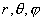), the gradient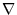is: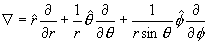The spherical wave equation is: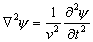Where ‘v’ is the speed of the wave which propagate through radial direction, for the special case in which it has angular symmetry (not dependent on the), then we will have the following wave equation: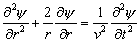Thus: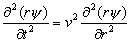Thus, we have the following general solution: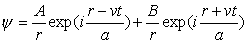Thus: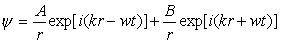In which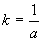Where k is the wave number.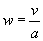Where w is the angular frequency.